# AV engines evasion techniques - part 4. Simple C++ example.

Hello, cybersecurity enthusiasts and white hackers!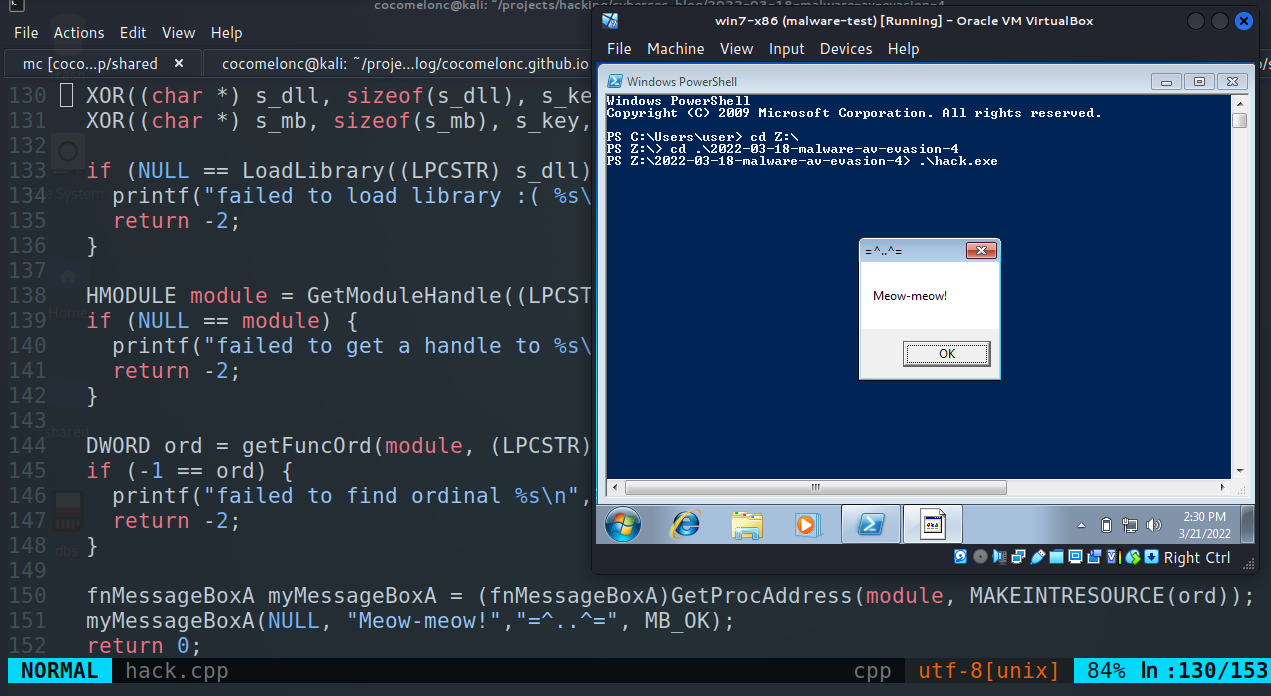This post is a result of my own research into another AV evasion trick. An example how to bypass AV engines in simple C++ malware.

This trick regarding how you hide your windows API calls from static analysis.

When you want to interact with the windows operating system, then you need to call windows API for example from `user32.dll` from your code such as `MessageBoxA` or any other API. If you specify the calls from your code, then the compiler will include the `MessageBoxA` or all the API’s needed in the import table in your PE. it would give ideas for the malware analyst for more closely investigate you malware.

### what is ordinals?

Each function exported by a DLL is identified by a numeric ordinal and optionally a name. Likewise, functions can be imported from a DLL either by ordinal or by name. The ordinal represents the position of the function’s address pointer in the DLL Export Address table.

In one of my previous posts I wrote a simple python script which enumerates the exported functions from the provided DLL (`dll-def.py`):

``````import pefile
import sys
import os.path

dll = pefile.PE(sys.argv)
dll_basename = os.path.splitext(sys.argv)

try:
with open(sys.argv.split("/")[-1].replace(".dll", ".def"), "w") as f:
f.write("EXPORTS\n")
for export in dll.DIRECTORY_ENTRY_EXPORT.symbols:
if export.name:
f.write('{}={}.{} @{}\n'.format(export.name.decode(), dll_basename, export.name.decode(), export.ordinal))
except:
print ("failed to create .def file :(")
else:
print ("successfully create .def file :)")
``````

Let’s go to run it for `user32.dll`:

``````python3 dll-def.py user32.dll
``````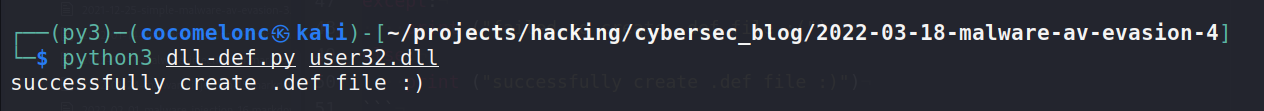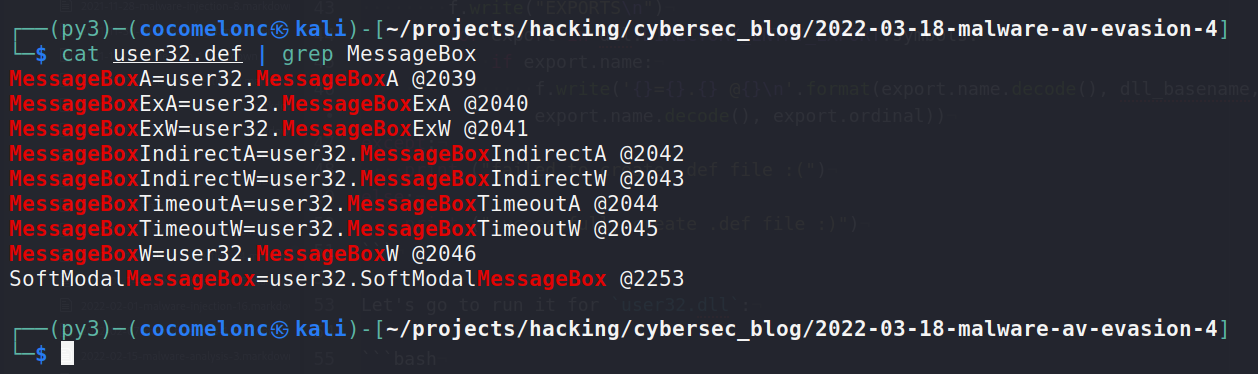As you can see, for example, for `MessageBoxA` ordinal is `2039`, for `MessageBoxW` ordinal is `2046`.

### practical example.

Let’s go to look at the practical example.

The ordinals might change on each release of the dll. We do not hardcode it in our code. We need to look up the ordinals by iterating the list and make a string comparison. This activity is counterproductive to our objective to hide the API name in our code since we need to make a string comparison during the lookup.

This technique is very simple.

First of all, I used a trick from my previous post:

``````// encrypted function name (MessageBoxA)
unsigned char s_mb[] = { 0x20, 0x1c, 0x0, 0x6, 0x11, 0x2, 0x17, 0x31, 0xa, 0x1b, 0x33 };

// encrypted module name (user32.dll)
unsigned char s_dll[] = { 0x18, 0xa, 0x16, 0x7, 0x43, 0x57, 0x5c, 0x17, 0x9, 0xf };

// key
char s_key[] = "mysupersecretkey";

// XOR decrypt
void XOR(char * data, size_t data_len, char * key, size_t key_len) {
int j;
j = 0;
for (int i = 0; i < data_len; i++) {
if (j == key_len - 1) j = 0;
data[i] = data[i] ^ key[j];
j++;
}
}

``````

And use python script to XOR encrypt our function name:

``````import sys
import os
import hashlib
import string

## XOR function to encrypt data
def xor(data, key):
key = str(key)
l = len(key)
output_str = ""

for i in range(len(data)):
current = data[i]
current_key = key[i % len(key)]
ordd = lambda x: x if isinstance(x, int) else ord(x)
output_str += chr(ordd(current) ^ ord(current_key))
return output_str

## encrypting
def xor_encrypt(data, key):
ciphertext = xor(data, key)
ciphertext = '{ 0x' + ', 0x'.join(hex(ord(x))[2:] for x in ciphertext) + ' };'
print (ciphertext)
return ciphertext, key

## key for encrypt/decrypt
my_secret_key = "mysupersecretkey"

ciphertext, p_key = xor_encrypt("user32.dll", my_secret_key)
ciphertext, p_key = xor_encrypt("MessageBoxA", my_secret_key)
``````

So in our case, we encrypt `user32.dll` and `MessageBoxA` strings.

In general, we use the Name Pointer Table (NPT) and Export Ordinal Table (EOT) to find export ordinals.

So I used function for get export directory table:

``````// get export directory table
PIMAGE_EXPORT_DIRECTORY getEDT(HMODULE module) {
PBYTE           base; // base address of module
PIMAGE_EXPORT_DIRECTORY edt;  // export directory table (EDT)
DWORD           rva;  // relative virtual address of EDT
PDWORD          sig;  // PE signature

// Start at the base of the module. The MS-DOS stub begins there.
base = (PBYTE)module;

// Get the PE signature and verify it.
if (IMAGE_NT_SIGNATURE != *sig) {
// Bad signature -- invalid image or module handle
return NULL;
}

// Get the COFF file header.

// Get the "optional" header (it's not actually optional for executables).

// Finally, get the export directory table.
// This image doesn't have an export directory table.
return NULL;
}
edt = (PIMAGE_EXPORT_DIRECTORY)(base + rva);

return edt;
}
``````

And searches a module’s name pointer table (NPT) for the named procedure:

``````// binary search
DWORD findFuncB(PDWORD npt, DWORD size, PBYTE base, LPCSTR proc) {
INT   cmp;
DWORD max;
DWORD mid;
DWORD min;

min = 0;
max = size - 1;

while (min <= max) {
mid = (min + max) >> 1;
cmp = strcmp((LPCSTR)(npt[mid] + base), proc);
// printf("check API name %s on %d\n", (LPCSTR)(npt[mid] + base), mid);

if (cmp < 0) {
min = mid + 1;
} else if (cmp > 0) {
max = mid - 1;
} else {
return mid;
}
}
return -1;
}
``````

As you can see, is simply a convenience function that does the binary search of the NPT.

Finally, get ordinal:

``````// get func ordinal
DWORD getFuncOrd(HMODULE module, LPCSTR proc) {
PBYTE           base; // module base address
PIMAGE_EXPORT_DIRECTORY edt;  // export directory table (EDT)
PWORD           eot;  // export ordinal table (EOT)
DWORD           i;  // index into NPT and/or EOT
PDWORD          npt;  // name pointer table (NPT)

base = (PBYTE)module;

// Get the export directory table, from which we can find the name pointer
// table and export ordinal table.
edt = getEDT(module);

// Get the name pointer table and search it for the named procedure.
i = findFuncB(npt, edt->NumberOfNames, base, proc);
if (-1 == i) {
// The procedure was not found in the module's name pointer table.
return -1;
}

// Get the export ordinal table.

// Actual ordinal is ordinal from EOT plus "ordinal base" from EDT.
return eot[i] + edt->Base;
}
``````

And `main` function idea without error checking:

``````int main(int argc, char* argv[]) {
XOR((char *) s_dll, sizeof(s_dll), s_key, sizeof(s_key));
XOR((char *) s_mb, sizeof(s_mb), s_key, sizeof(s_key));
HMODULE module = GetModuleHandle((LPCSTR) s_dll);
DWORD ord = getFuncOrd(module, (LPCSTR) s_mb);
myMessageBoxA(NULL, "Meow-meow!","=^..^=", MB_OK);
return 0;
}
``````

So the full source code of our example:

``````/*
* hack.cpp - Find function from DLL via ordinal. C++ implementation
* @cocomelonc
* https://cocomelonc.github.io/tutorial/2022/03/18/simple-malware-av-evasion-4.html
*/
#include <stdio.h>
#include "windows.h"

typedef UINT(CALLBACK* fnMessageBoxA)(
HWND   hWnd,
LPCSTR lpText,
LPCSTR lpCaption,
UINT   uType
);

// encrypted function name (MessageBoxA)
unsigned char s_mb[] = { 0x20, 0x1c, 0x0, 0x6, 0x11, 0x2, 0x17, 0x31, 0xa, 0x1b, 0x33 };

// encrypted module name (user32.dll)
unsigned char s_dll[] = { 0x18, 0xa, 0x16, 0x7, 0x43, 0x57, 0x5c, 0x17, 0x9, 0xf };

// key
char s_key[] = "mysupersecretkey";

// XOR decrypt
void XOR(char * data, size_t data_len, char * key, size_t key_len) {
int j;
j = 0;
for (int i = 0; i < data_len; i++) {
if (j == key_len - 1) j = 0;
data[i] = data[i] ^ key[j];
j++;
}
}

// binary search
DWORD findFuncB(PDWORD npt, DWORD size, PBYTE base, LPCSTR proc) {
INT   cmp;
DWORD max;
DWORD mid;
DWORD min;

min = 0;
max = size - 1;

while (min <= max) {
mid = (min + max) >> 1;
cmp = strcmp((LPCSTR)(npt[mid] + base), proc);
// printf("check API name %s on %d\n", (LPCSTR)(npt[mid] + base), mid);

if (cmp < 0) {
min = mid + 1;
} else if (cmp > 0) {
max = mid - 1;
} else {
return mid;
}
}
return -1;
}

// get export directory table
PIMAGE_EXPORT_DIRECTORY getEDT(HMODULE module) {
PBYTE           base; // base address of module
PIMAGE_EXPORT_DIRECTORY edt;  // export directory table (EDT)
DWORD           rva;  // relative virtual address of EDT
PDWORD          sig;  // PE signature

// Start at the base of the module. The MS-DOS stub begins there.
base = (PBYTE)module;

// Get the PE signature and verify it.
if (IMAGE_NT_SIGNATURE != *sig) {
// Bad signature -- invalid image or module handle
return NULL;
}

// Get the COFF file header.

// Get the "optional" header (it's not actually optional for executables).

// Finally, get the export directory table.
// This image doesn't have an export directory table.
return NULL;
}
edt = (PIMAGE_EXPORT_DIRECTORY)(base + rva);

return edt;
}

// get func ordinal
DWORD getFuncOrd(HMODULE module, LPCSTR proc) {
PBYTE           base; // module base address
PIMAGE_EXPORT_DIRECTORY edt;  // export directory table (EDT)
PWORD           eot;  // export ordinal table (EOT)
DWORD           i;  // index into NPT and/or EOT
PDWORD          npt;  // name pointer table (NPT)

base = (PBYTE)module;

// Get the export directory table, from which we can find the name pointer
// table and export ordinal table.
edt = getEDT(module);

// Get the name pointer table and search it for the named procedure.
i = findFuncB(npt, edt->NumberOfNames, base, proc);
if (-1 == i) {
// The procedure was not found in the module's name pointer table.
return -1;
}

// Get the export ordinal table.

// Actual ordinal is ordinal from EOT plus "ordinal base" from EDT.
return eot[i] + edt->Base;
}

int main(int argc, char* argv[]) {
XOR((char *) s_dll, sizeof(s_dll), s_key, sizeof(s_key));
XOR((char *) s_mb, sizeof(s_mb), s_key, sizeof(s_key));

if (NULL == LoadLibrary((LPCSTR) s_dll)) {
printf("failed to load library :( %s\n", s_dll);
return -2;
}

HMODULE module = GetModuleHandle((LPCSTR) s_dll);
if (NULL == module) {
printf("failed to get a handle to %s\n", s_dll);
return -2;
}

DWORD ord = getFuncOrd(module, (LPCSTR) s_mb);
if (-1 == ord) {
printf("failed to find ordinal %s\n", s_mb);
return -2;
}

myMessageBoxA(NULL, "Meow-meow!","=^..^=", MB_OK);
return 0;
}
``````

### demo

Let’s go to compile our example:

``````i686-w64-mingw32-g++ hack.cpp -o hack.exe -mconsole -I/usr/share/mingw-w64/include/ -s -ffunction-sections -fdata-sections -Wno-write-strings -Wint-to-pointer-cast -fno-exceptions -fmerge-all-constants -static-libstdc++ -static-libgcc -fpermissive
``````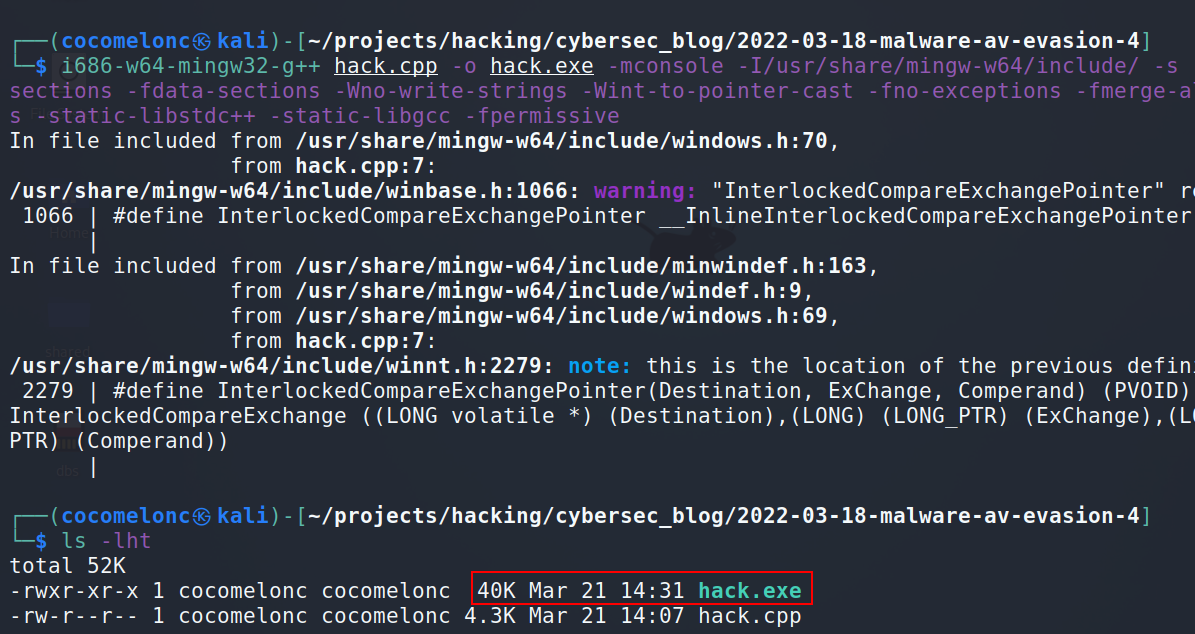And run:

``````.\hack.exe
``````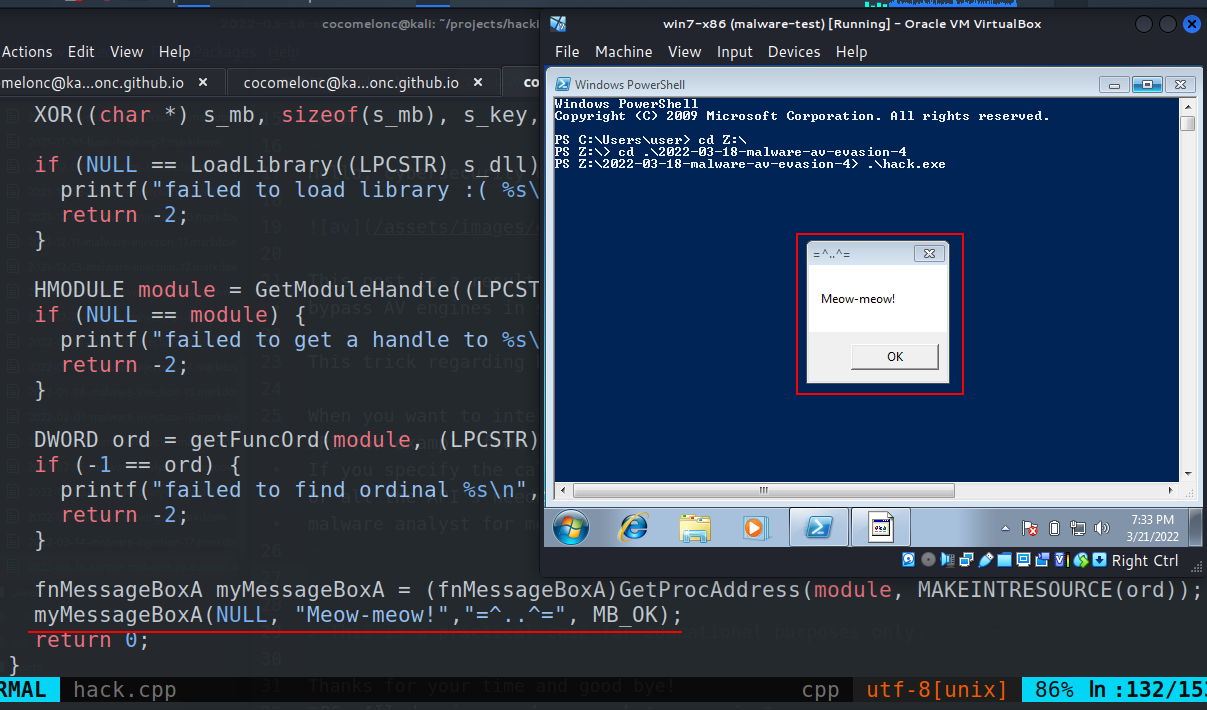As you can see, everything is work perfectly, for purity of the experiment I add one line to my `hack.cpp` in `main` function:

``````//..
DWORD ord = getFuncOrd(module, (LPCSTR) s_mb);
if (-1 == ord) {
printf("failed to find ordinal %s\n", s_mb);
return -2;
}
printf("MessageBoxA ordinal is %d\n", ord);
//..
``````

Compile and run: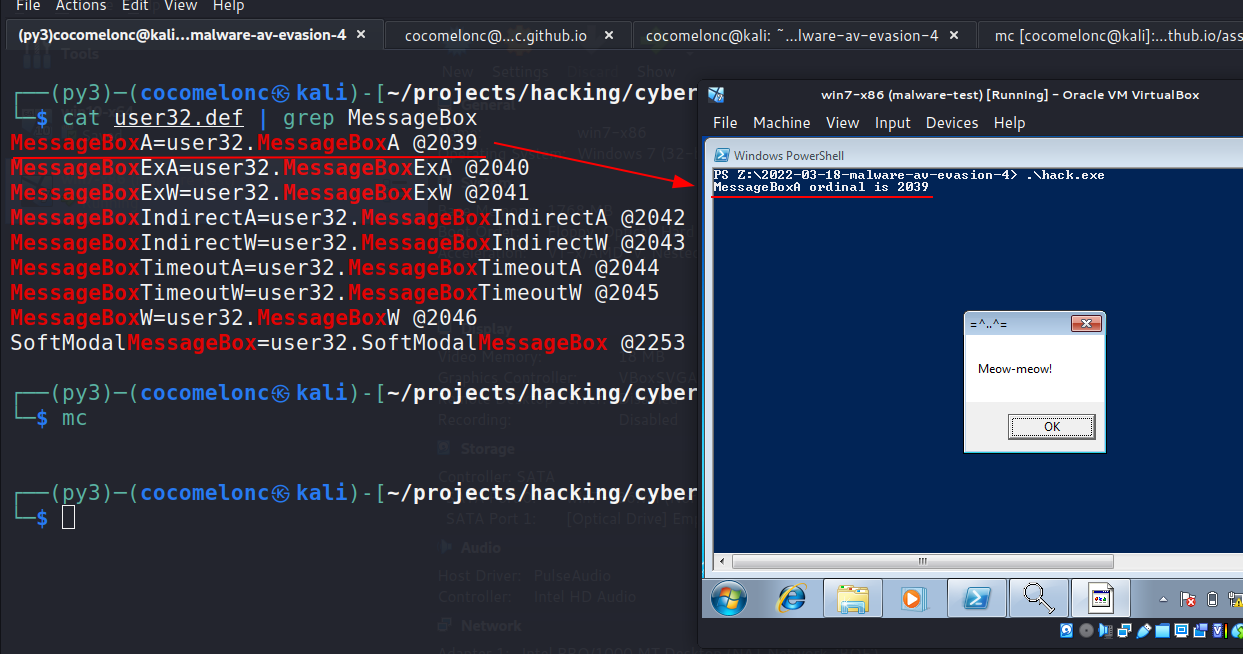As you can see, our malware successfully find correct ordinal. Perfect :)

String search result:

``````strings -n 8 hack.exe | grep MessageBox
``````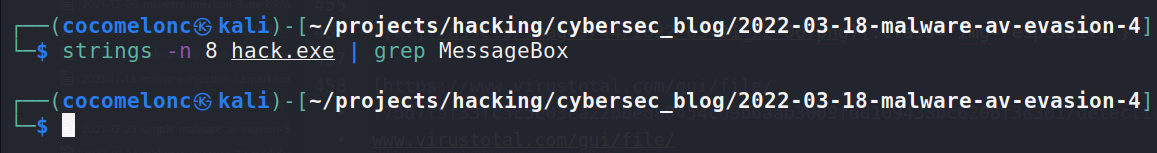As you can see no `MessageBox` in strings check. So this is how you hide your windows API calls from static analysis.

Let’s go to upload to VirusTotal: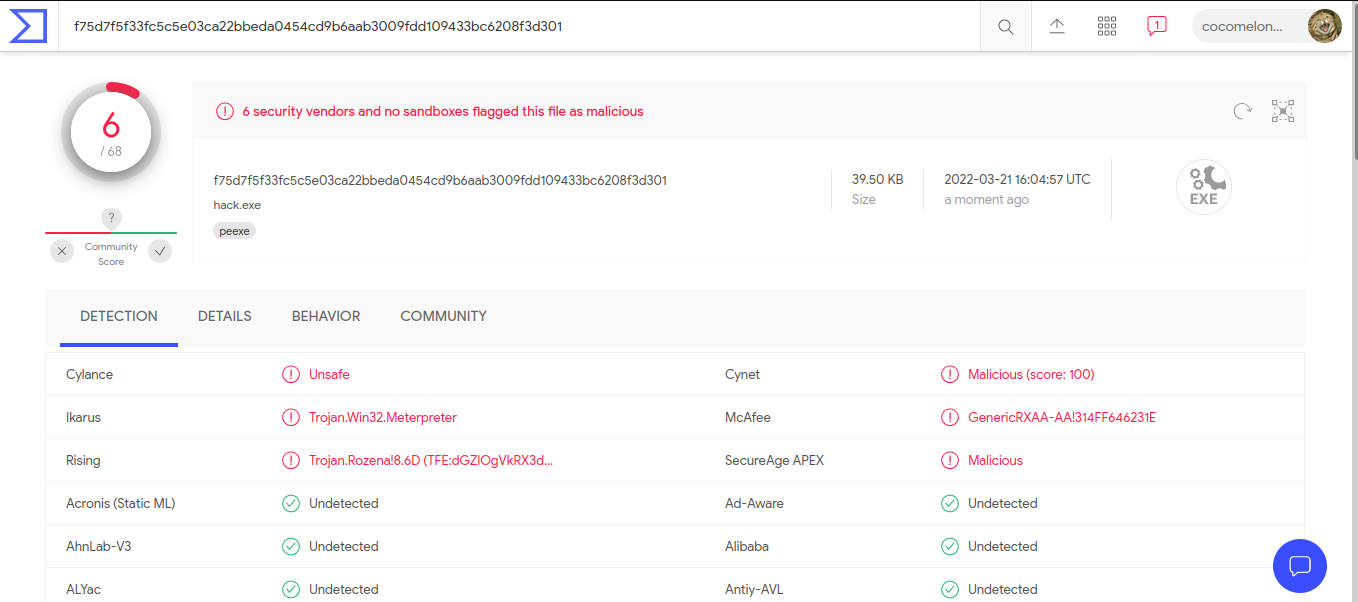https://www.virustotal.com/gui/file/f75d7f5f33fc5c5e03ca22bbeda0454cd9b6aab3009fdd109433bc6208f3d301/detection

So 6 of 68 AV engines detect our file as malicious

I hope this post spreads awareness to the blue teamers of this interesting technique, and adds a weapon to the red teamers arsenal.

This is a practical case for educational purposes only.

Thanks for your time and good bye!
PS. All drawings and screenshots are mine

Tags:

Categories:

Updated: